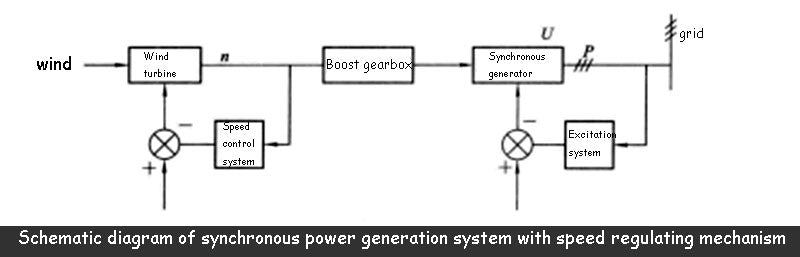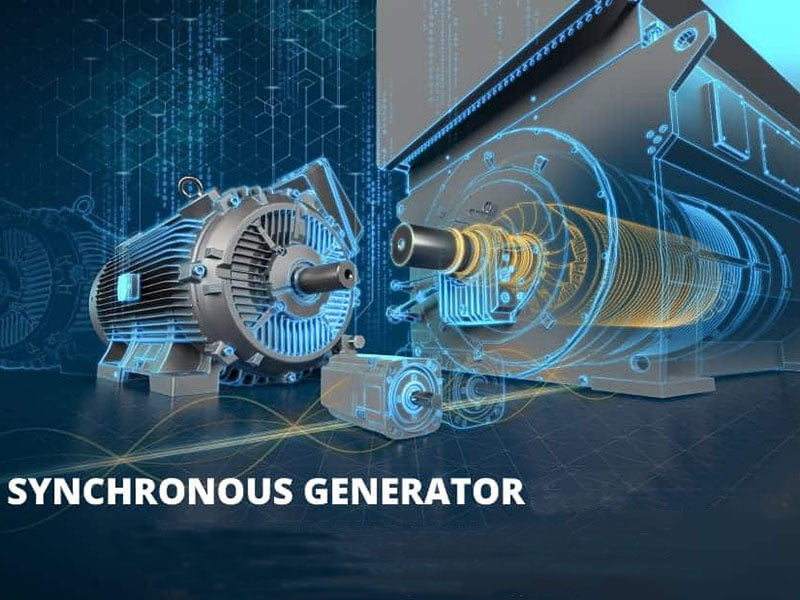## 1. Synchronous generator grid connection method

### 1.1 The synchronous generator is automatically quasi-synchronous and connected to the grid

In the conventional grid-connected power generation system, the synchronous generator using three-phase windings is the most common. The synchronous generator can output both active power and reactive power during operation, and the frequency of the synchronous generator is stable and the power quality is high. , so the synchronous generator is widely accepted by the power system. In a synchronous generator, there is a strictly invariable fixed relationship between the number of pole pairs, speed and frequency of the generator, that is,For a synchronous generator to be connected to the grid via the standard synchronous grid connection method, the following four conditions must be met:

① The voltage of the synchronous generator is equal to the voltage of the grid, and the voltage waveform is the same.

② The voltage phase sequence of the synchronous generator is the same as the voltage phase sequence of the power grid.

③ The frequency f s of the synchronous generator is the same as the frequency f1 of the grid.

④ The voltage phase angle of the synchronous generator at the moment of the parallel gate is consistent with the parallel phase angle of the power grid.

The figure below shows the parallel connection between the synchronous generator driven by the wind turbine and the power grid. In the figure, UAB, U Bc, and UAC are the grid voltage; UABs, UBCs, and UcAs are the generator voltage; nT is the wind turbine speed; ns is the generator speed.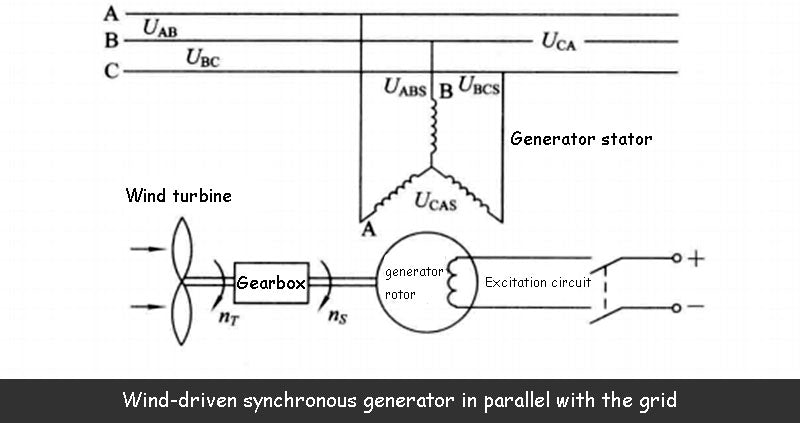The rotating shaft of the wind turbine and the rotating shaft of the synchronous generator are connected by a speed-up gear and a coupling.

The grid connection method that satisfies the above ideal grid connection conditions is the quasi-synchronous grid connection method. Under this grid connection condition, there will be no inrush current generated at the moment of grid connection, and the grid voltage will not drop, and it will not affect the synchronous generator. Damage to stator windings and other mechanical parts. This is the biggest advantage of this grid-connected method, but for wind-driven synchronous generator, it is actually not easy to accurately achieve this ideal grid-connected condition. In the actual grid-connected operation, the voltage, frequency and phase There are often some deviations, so there will still be some inrush currents when connected to the grid. It is generally stipulated that the voltage difference between the synchronous generator and the power grid system does not exceed 5%~10%, and the frequency difference does not exceed 0.1%~0.5%, so that the inrush current does not exceed its allowable range. However, if the voltage and frequency of the power grid itself often fluctuate greatly, it will be more difficult to achieve quasi-synchronous grid connection by synchronous generator in one step.

### 1.2 The synchronous generator is self-synchronized and connected to the grid

Self-synchronized grid connection means that the synchronous generator is driven by the prime mover when the rotor is not excited and the excitation winding is short-circuited by the current limiting resistor. % synchronous speed), put the synchronous generator into the power grid, and then immediately put into excitation, relying on the action of the electromagnetic force between the stator and the rotor of the synchronous generator, the synchronous generator automatically pulls people to run synchronously. Since the synchronous generator is not excited when it is put into the grid, there is no full-step process of adjusting and calibrating the generator voltage and phase angle when the quasi-synchronous grid is connected, and the possibility of asynchronous closing is fundamentally excluded. sex. This grid-connected method is often used when the synchronous generator needs to be quickly put into parallel operation after the power grid fails and returns to normal. The advantage of this grid-connected method is that it does not require complicated grid-connected devices, the grid-connected operation is simple, and the grid-connected process is fast; The current when the three phases of the generator output are suddenly short-circuited), the grid voltage will drop for a short time. The degree of grid voltage reduction and the length of the voltage recovery time are related to the ratio of the capacity of the synchronous generator to the grid capacity, and in the case of wind power generation, it is also related to the wind resource characteristics of the wind farm.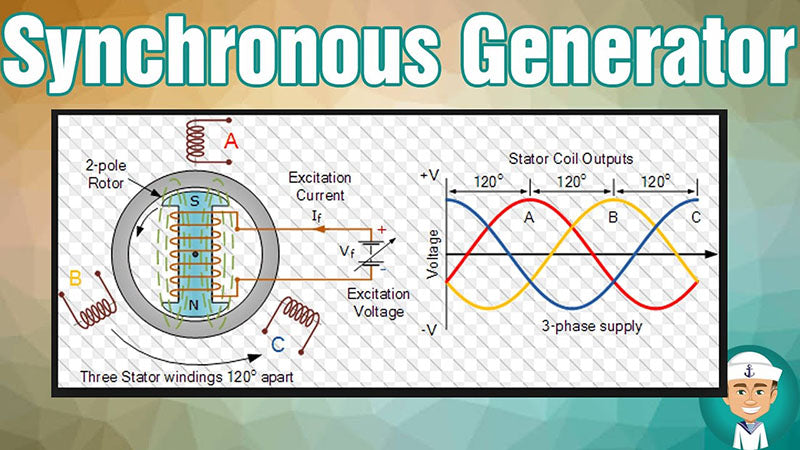It must be pointed out that the self-synchronization process of synchronous generator is closely related to the time of excitation and the rate of excitation growth after excitation. If the synchronous generator is put into the power grid when it is very close to the synchronous speed, the excitation should be added quickly to ensure that the synchronous generator can be quickly pulled into synchronization, and the greater the rate of excitation growth, the self-synchronization process of the synchronous generator will end. The faster it is, but when the speed of the synchronous generator is larger than the synchronous speed, it should be avoided to put into excitation immediately, otherwise the synchronous generator will generate a large synchronous torque and cause a large oscillating current during the self-synchronization process of the synchronous generator. and torque.

## 2. Torque-speed characteristics of synchronous generator

When the synchronous generator runs normally after being connected to the grid, its torque-speed characteristic curve is shown in the figure below, where ns is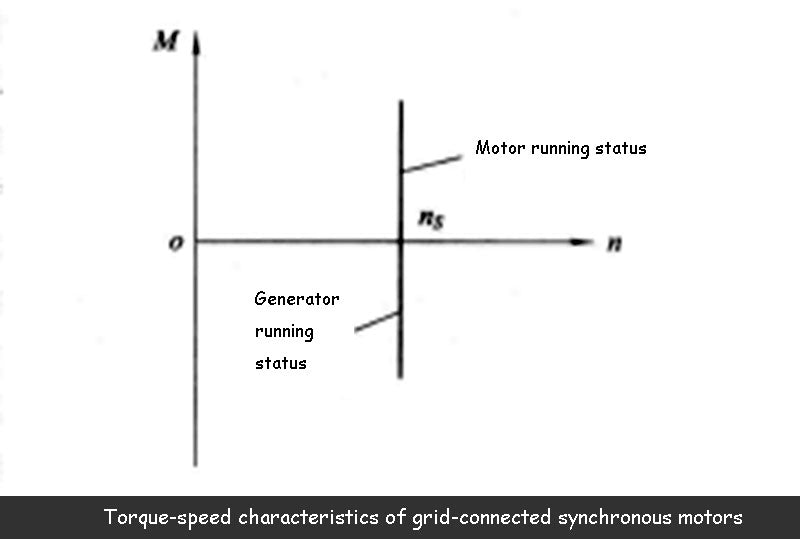The synchronous speed of the synchronous generator. As can be seen from the above figure, the electromagnetic torque of the synchronous generator is a braking torque property for the wind turbine. Therefore, no matter how the electromagnetic torque changes, the speed of the synchronous generator should remain unchanged. (i.e. maintained at synchronous speed ns) in order to maintain the frequency of the synchronous generator to be the same as the frequency of the grid, otherwise the synchronous generator will be disconnected from the grid. This requires the wind turbine to have an accurate speed regulating mechanism. When the wind speed changes, the speed of the synchronous generator can be maintained unchanged, which is equal to the synchronous speed. The operation mode of this wind power generation system is called constant speed and constant frequency mode. Correspondingly, under the operation mode of the variable speed constant frequency system (that is, the speed of the wind turbine and the synchronous generator varies with the wind speed, and the output end of the synchronous generator can still obtain the power output equal to the grid frequency), Wind turbines do not need a speed regulating mechanism. The schematic block diagram of a synchronous wind power generation system with a speed regulating mechanism is shown in the figure below.

The speed control system is used to control the speed of the wind turbine (that is, the speed of the synchronous generator) and the active power. The excitation system is used to control the voltage and reactive power of the synchronous generator. In the figure, n, U, and P represent the speed of the wind turbine respectively. , generator voltage, output power. In short, after the synchronous generator is connected to the grid, the voltage, frequency and output power of the generator must be effectively controlled, otherwise the phenomenon of loss of synchronization will occur.